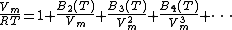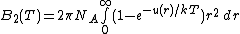# Virial equation

## Virial equation

An equation of state of gases that has additional terms beyond that for an ideal gas, which account for the interactions between the molecules. The pressure p can be expressed in terms of the molar volume Vm = V/n (where n is the number of moles of gas molecules in a volume V), the absolute temperature T, and the universal gas constant R = 8.3145 J K-1 mol-1 or, in more commonly used practical units, 0.082058 L atm K-1 mol-1 [Eq. (1)].

(1)In the equation the virial coefficients Bn(T) are functions only of the temperature and depend on the nature of the gas. In an ideal gas, in which all interactions between the molecules can be neglected because Vm is sufficiently large, only the first term, unity, survives on the right-hand side.

The equation is important because there are rigorous relations between the coefficients B2, B3, and so on, as well as the interactions of the molecules in pairs, triplets, and so forth. It provides a valuable route to a knowledge of the intermolecular forces. Thus if the intermolecular energy of a pair of molecules at a separation r is u(r), then the second virial coefficient can be expressed as Eq. (2).

(2)Here NA = 6.0221 × 1023 mol-1 is the Avogadro constant, and k = R/NA. In a gas mixture, Bn is a polynomial of order n in the mole fractions of the components. See Intermolecular forces

The virial equation is useful in practice because it represents the pressure accurately at low and moderate gas densities, for example, up to about 4 mol L-1 for nitrogen at room temperature, which corresponds to a pressure of about 100 atm (10 MPa). It is not useful at very high densities, where the series may diverge, and is inapplicable to liquids. It can be rearranged to give the ratio pVm/RT as an expansion in powers of the pressure instead of the density, which is equally useful empirically, but the coefficients of the pressure expansion are not usually called virial coefficients, and lack any simple relation to the intermolecular forces or, in a mixture, to the composition of the gas. See Van der Waals equation

McGraw-Hill Concise Encyclopedia of Physics. © 2002 by The McGraw-Hill Companies, Inc.
Mentioned in ?
References in periodicals archive ?
They evolved from the virial equation of state and became common when pure fluids had been characterized over wide ranges of states and in multiple properties because all this information could be represented with high accuracy in a single formulation of the Helmholtz energy.
Summary: A virial equation of state was applied to oxygen spectroscopic measurements at 1270 nm band.
Kamerlingh-Omnes [10,11] was proposed a virial equation of state for real gases in Z as an infinite power series(The virial expansion) in terms of molar density (Eq.) for a pure gas:
The theoretical basis in statistical mechanics for the virial equation is one of its attractions.
This temperature-correction parameter was also proved in developing a new virial equation of state by Ichikura et al.
The mixture moist air is calculated using the following mixing virial equation of state.
This real gas model is based on the use of a virial equation of state for specific volume, Beattie's formulation (Beattie 1949) for enthalpy and entropy, and the so-called enhancement factor equation for the interaction of air-water vapor molecules (Hyland and Wexler 1973a,1973b; Hyland 1975).
Effect of Non-Ideal Gas Behaviour via the Virial Equation of State on Dufour Conduction in Macroporous Catalysts
"The proposed model consists of the PR equation for the VLE behavior, which has to be combined with the truncated virial equation of state for the vapor phase of these mixtures with different compositions," they explained.
Often, the virial equation is written in the form of an expression for the pressure as a sum of the powers of density multiplied by temperature-dependent virial coefficients.
The atomic density was determined using the virial equation up to the third virial coefficient.
Mason and Spurling noted the special importance of the virial equation of state because of its sound theoretical foundation (21).

Site: Follow: Share:
Open / Close